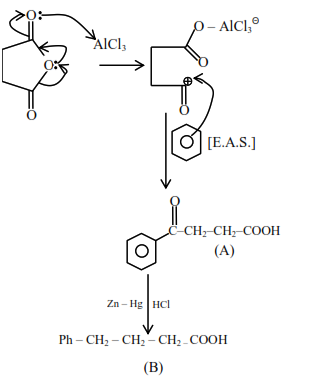# Solve this followingQuestion:

The structures of $\mathbf{A}$ and $\mathbf{B}$ formed in the following reaction are: $\left[\mathrm{Ph}=-\mathrm{C}_{6} \mathrm{H}_{5}\right]$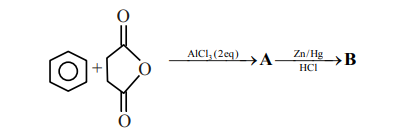1.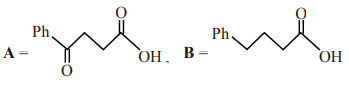2.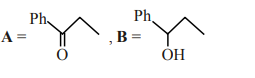3.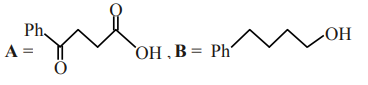4.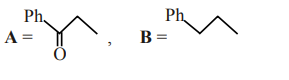Correct Option: 1

Solution: